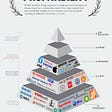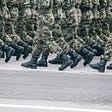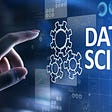# Data Pre-processing-Refining Gold

“Data is the new currency” ……more precisely “Usable Data is the new Currency” don’t get the difference? let me give you an example: — imagine you want to make a New York-style pizza (yeah, I love pizza too). So, you go to a grocery store to buy the ingredients and see a ton of ingredients in front of you but obviously, you don’t need to buy the entire store to make a single pizza so you google the ingredients to make a pizza umm… Let’s say pizza dough, tomatoes, pepperoni & cheese LOTS OF CHEESE then you take the ingredients to the cashier and then head home and make the most delicious pizza in the history of mankind. The store is the entire data available; the ingredients are the data that you actually need to make the pizza & the process of separating the usable data from the entire data set is called “Data Pre-processing”

Now, let’s go a step further there is a possibility that the data which you have might still be missing some values or in simple words just not ready for execution, for example, you have just bought a new wooden pencil and that is what you need but you can’t use it straight off you will need to sharpen the pencil in order to use it. When you take up a data set you will need to ready the data in order to use it, it can have various issues like missing data, inconsistent data, different categorical data etc. This will increase the QUALITY of your data this process is also called “Data Cleaning”. It is said data scientists spend 90% of their time doing just that data cleaning

Now that we have some understanding of what data pre-processing and data cleaning is let us dive into some technicalities.

It takes 7 steps in order to get your data ready for use: -

1. Data Acquisition (Ingredients)
2. Importing the required libraries (Kitchen)
3. Importing the Data Set (Kitchen ingredients)
4. Handling the missing data (Checking the ingredients)
5. Encoding the categorical data (Do all ingredients go together?)
6. Splitting the data set
7. Feature Scaling (Setting up all the ingredients on the dough)

## Data Acquisition

There are two methods for anyone to acquire data either you collect the data manually or you use websites like Kaggle or UCI which have a lot of data sets available from various fields but whichever of these two ways you use it should be in CSV, or HTML, or XLSX file formats.

You can click here to see a very small example of a data set. Suppose we need to predict if people have purchased a commodity or not (in the above data set), depending on where they are from, age and salary. The result purchased is the dependent variable as it depends on where they are from, age and salary, these three are the independent variables.

Importing the required libraries

Python is the most preferred and most used language by data scientists. It requires us to import certain libraries in order to do data science efficiently, these libraries help in pre-processing in machine learning. These are the three fundamental libraries of python used in data pre-processing: -

1.NumPy-It’s the Einstein of python. You want any scientific calculation done in python call NumPy. Using NumPy you can add multidimensionally arrays and matrices to the code (example: -data set above). To add NumPy to your code there are several ways for some IDE’s you require to install the NumPy separately and some may have it built-in like in google collab.

In order to import the library, you need to type “import numpy” and the library will be imported. This library will be called several times so we can assign a short form as “np”.

`import numpy as np`

2.Pandas-Data manipulation and analysis is the forte of pandas. It has high performance, easy to use data structures and data analysis for python. It is also used extensively so it is usually preferred to assign a short form as “pd”

`import pandas as pd`

3. Matplotlib-All graphs or any sort of plotting in python is done using this library. It is capable of creating any plot using the mathematical equations. In data science we can derive a lot of information from the plot of an expression. We can create a short form of it as “plt”

`import matplotlib.pyplot as plt`

Importing the data set

Our kitchen is ready, now time to bring in the ingredients. Here first you need to add the data set or import it, in different Ide’s there are different methods to add the data set to your ide some require many steps some require minimum. I would suggest using google collab as it requires the minimum steps.

After uploading the data set you need to let your code know which data set you are using so you use a feature of panda called “read_csv” to detect the data set.

Now after letting your code know which data set you are using you need to specify which columns in your data set will be independent variables and which columns will be dependent variables. This can be done using the “iloc[]” feature of pandas.

`dataset=pd.read_csv('Data.csv')X=dataset.iloc[:, :-1].valuesY=dataset.iloc[:, -1].values`

Here, for X the first “:” is to include all the rows and after comma “: -1” is done to tell the code to select all columns except the last one which contains the dependent variable. for Y first “:” is for selecting all rows and -1 is to select only the row containing the dependent variable.

Handling the missing data

Now at times the data which we have is inconsistent or in simpler terms misses a few data pieces or data entries. This issues can be solved by two methods either by removing the entire row that contains the missing data or taking the average or mean of that particular row and filling the spaces with that value.

`from sklearn.impute import SimpleImputerImputer= SimpleImputer(missing_values=np.nan, strategy='mean')Imputer.fit(X[:, 1:3])X[:, 1:3]=Imputer.transform(X[:, 1:3])`

Remember Pandas, NumPy and matplotlib, sklearn one such library which helps us take care of the missing data in our data set. Above is an example code for such a missing data issue using by taking the mean of that row.

Encoding the categorical data

Real-life data sets at times, data available is not just in numbers sometimes it is in terms of alphabets. Remember the data set which we used above it has the option of countries which contain country names which is not as same as the rest of the data which is in number format so……Yeah you guessed it we convert it to numbers as well, now which numbers to convert it into is another question that comes in mind. In order to keep things simple, we convert them into a different combination of 1s and 0s and in to do that we remove all the countries from a single column and assign them their own respective columns. Then provide them with 1s and 0s in a way that if it belongs to the country it will be given 1 and if it does not belong to the country it will be assigned 0.

A little too much work? Don’t worry there is a reason we use soo many imported libraries they will do this work for us. All this is done by using the “OneHotEncoder” present in the sklearn library. It divides our data set and “LabelEncoder” labels it.

`from sklearn.compose import ColumnTransformerfrom sklearn.preprocessing import OneHotEncoderct = ColumnTransformer(transformers=[('encoder',OneHotEncoder(),)],remainder='passthrough')X=np.array(ct.fit_transform(X))from sklearn.preprocessing import LabelEncoderle = LabelEncoder()Y = le.fit_transform(Y)`

Here is the code Enjoy!

Now we are at the stage where we need to check if our data is correct or not and to do that, we need different machine learning models but don’t worry we won’t be getting into ML in this blog(phew). Before putting the data set into an ML model, we need to split our data into “training set” and “test set”. Let’s keep the ratio as 80% to 20% since we need to train more in comparison to the test.

`from sklearn.model_selection import train_test_splitX_train, X_test, y_train, y_test = train_test_split(X, y, test_size = 0.2, random_state = 1)`

Here we call on to sklearn AGAIN to help us split the data.

• x_train — features for the training data
• x_test — features for the test data
• y_train — dependent variables for training data
• y_test — independent variable for testing data

Feature Scaling

THE FINAL STAGE

Since we have a large data set at times our data is not on the same scale. Some may be in 1s and 0s and some may be in 10s of zeros so this would definitely contaminate our data set when we use it for anything actually either just ML or entire data science. So, we bring our entire data set to the same scale.

Take a wild guess which library we will be using…. YES, its sklearn YET AGAIN

`from sklearn.preprocessing import StandardScalersc = StandardScaler()X_train[:, 3:] = sc.fit_transform(X_train[:, 3:])X_test[:, 3:] = sc.transform(X_test[:, 3:])`

Q)Should we apply feature scaling to the training set or the test set or both?

Remember we split our data into a training set and a test set. We won’t be feature scaling all of them together because that would contaminate our test set. But yet again we will have to scale it because our test set is on a different scale when compared to our training set after feature scaling it so…. we use the mean of our training set to scale the test set.

So, now you know how making a pizza can help you understand data pre-processing😄

Here are a few sources for further or more in-depth study

Source 1

Source 2

If you liked this blog or are in general a data scientist enthusiast feel free to mail me at aishwar99govil@gmail.com or connect with me on Instagram -aishwargovil

--

--

--

## More from The Startup

Get smarter at building your thing. Follow to join The Startup’s +8 million monthly readers & +756K followers.

## Statistics Part II: Bayesian To The Rescue## Publish Data Outside Your Data Lake With a Spark Connector## Maslow’s Hierarchy of Profitability — Psyda’s Infographic adapted from Statista## 6 Questions to Ask Before Committing to a Bootcamp## U.S. Road Accidents Data Analysis and Visualization## Week 1 — Topify## No Experience? No Problem! Here’s How to Become a Data Scientist in 24 Weeks## The Most Efficient Way To Learn Data Analysis in 12 Weeks: Step By Step Guide## Aishwar Govil

Data Science || Machine Learning || IOT || Artificial Intelligence

## Market Basket Analysis — How Do I Know What Products Together?## How to Build a Complete Classification Model in R and caret## Finding Data Correlations